# [GSP245] Scikit-learn Model Serving with Online Prediction Using Cloud Machine Learning Engine

Делаю:
01.08.2019

### Overview

If you’ve built machine learning models with scikit-learn, and you want to serve your models in real time for an application, perhaps managing the resulting infrastructure sounds like a nightmare. Fortunately, there’s an alternative - serving your trained scikit-learn models on Cloud Machine Learning Engine (ML Engine).

You can now upload a model you’ve already trained onto Google Cloud Storage and use ML Engine’s online prediction service to support scalable prediction requests against your trained model.

In this lab you’ll learn how to train a simple scikit-learn model, upload the model to ML Engine, and make online predictions against that model.

### How to bring a scikit-learn model to ML Engine

Getting your model ready for prediction can be done in 5 steps:

1. Create and save a model to a file
3. Create a model resource on ML Engine
5. Make an online prediction

This lab will walk you through the five steps listed above.

### What you will build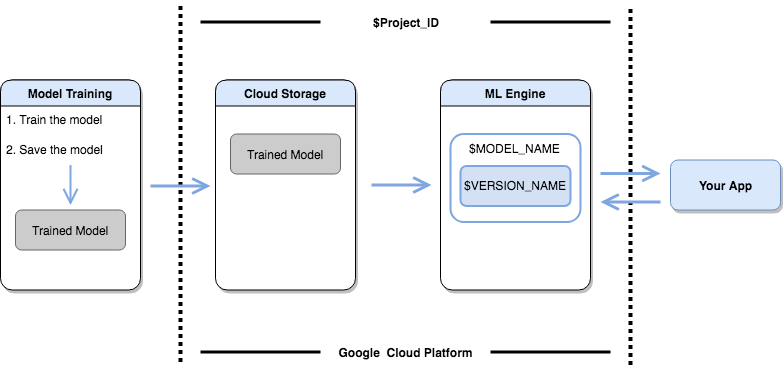### What you’ll learn

• How to create a model on ML Engine
• How to make online predictions against your model on ML Engine

### Create a storage bucket

$export PROJECT_ID=$(gcloud config list project --format "value(core.project)")
$export BUCKET_NAME=${PROJECT_ID}-bucket
$export REGION=us-central1  // Create the new bucket:$ gsutil mb -l $REGION gs://$BUCKET_NAME


$export MODEL_PATH=gs://$BUCKET_NAME


### Install scikit-learn

// Create isolated Python environment:
$virtualenv ml-env -p python3.5 // Activate virtualenv$ source ml-env/bin/activate

${ pip install google-api-python-client==1.6.2 pip install scikit-learn==0.19.1 pip install pandas==0.22.0 pip install scipy==1.0.0 }  ### Set up environment variables You’ll next set up these environment variables in order to run subsequent steps. • PROJECT_ID - Use the PROJECT_ID that matches your GCP project. • MODEL_PATH - The path to your model on GCS. • MODEL_NAME - Use your own model name, such as ‘census’. • VERSION_NAME - Use your own version, such as ‘v1’. • REGION- Select a region here or use the default ‘us-central1’; this is the region where the model will be deployed. • INPUT_DATA_FILE- Include a JSON file that contains the data used as input to your model’s predict method. (For more info see the complete guide.) Replace the lines below with the names of your variables. $ {
export MODEL_NAME=census
export VERSION_NAME=v1
export REGION=us-central1
}



### The data for this lab

The < a href=”https://archive.ics.uci.edu/ml/datasets/Census+Income”>Census Income Data Set</a> that this sample uses for training is hosted by the UC Irvine Machine Learning Repository. Citation: Dua, D. and Karra Taniskidou, E. (2017). UCI Machine Learning Repository. Irvine, CA: University of California, School of Information and Computer Science.

Create a directory to hold the data:

$mkdir census_data  Download the data you’ll use for this lab: $ curl https://archive.ics.uci.edu/ml/machine-learning-databases/adult/adult.data --output census_data/adult.data

$curl https://archive.ics.uci.edu/ml/machine-learning-databases/adult/adult.test --output census_data/adult.test  ### Train and save your model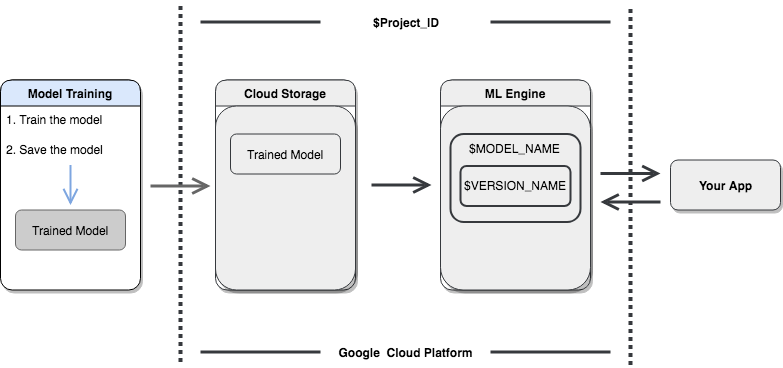Load the data into a pandas DataFrame to prepare it for use with XGBoost. Train a simple model in XGBoost. Save the model to a file that can be uploaded to Cloud ML Engine. First, we need to create a scikit-learn model and train it. Once we’ve done that, you can save your model and put it in a format that is usable by ML Engine. $ vi train.py

import googleapiclient.discovery
import json
import numpy as np
import os
import pandas as pd
import pickle
from sklearn.ensemble import RandomForestClassifier
from sklearn.externals import joblib
from sklearn.feature_selection import SelectKBest
from sklearn.pipeline import FeatureUnion
from sklearn.pipeline import Pipeline
from sklearn.preprocessing import LabelBinarizer

# Define the format of your input data including unused columns (These are the columns from the census data files)
COLUMNS = (
'age',
'workclass',
'fnlwgt',
'education',
'education-num',
'marital-status',
'occupation',
'relationship',
'race',
'sex',
'capital-gain',
'capital-loss',
'hours-per-week',
'native-country',
'income-level'
)

# Categorical columns are columns that need to be turned into a numerical value to be used by scikit-learn
CATEGORICAL_COLUMNS = (
'workclass',
'education',
'marital-status',
'occupation',
'relationship',
'race',
'sex',
'native-country'
)

# Load the training census dataset

# Remove the column we are trying to predict ('income-level') from our features list
# Convert the Dataframe to a lists of lists
train_features = raw_training_data.drop('income-level', axis=1).as_matrix().tolist()
# Create our training labels list, convert the Dataframe to a lists of lists
train_labels = (raw_training_data['income-level'] == ' >50K').as_matrix().tolist()

# Load the test census dataset
# Remove the column we are trying to predict ('income-level') from our features list
# Convert the Dataframe to a lists of lists
test_features = raw_testing_data.drop('income-level', axis=1).as_matrix().tolist()
# Create our training labels list, convert the Dataframe to a lists of lists
test_labels = (raw_testing_data['income-level'] == ' >50K.').as_matrix().tolist()

# Since the census data set has categorical features, we need to convert
# them to numerical values. We'll use a list of pipelines to convert each
# categorical column and then use FeatureUnion to combine them before calling
# the RandomForestClassifier.
categorical_pipelines = []

# Each categorical column needs to be extracted individually and converted to a numerical value.
# To do this, each categorical column will use a pipeline that extracts one feature column via
# SelectKBest(k=1) and a LabelBinarizer() to convert the categorical value to a numerical one.
# A scores array (created below) will select and extract the feature column. The scores array is
# created by iterating over the COLUMNS and checking if it is a CATEGORICAL_COLUMN.
for i, col in enumerate(COLUMNS[:-1]):
if col in CATEGORICAL_COLUMNS:
# Create a scores array to get the individual categorical column.
# Example:
#  data = [39, 'State-gov', 77516, 'Bachelors', 13, 'Never-married', 'Adm-clerical',
#         'Not-in-family', 'White', 'Male', 2174, 0, 40, 'United-States']
#  scores = [0, 1, 0, 0, 0, 0, 0, 0, 0, 0, 0, 0, 0, 0]
#
# Returns: [['Sate-gov']]
scores = []
# Build the scores array
for j in range(len(COLUMNS[:-1])):
if i == j: # This column is the categorical column we want to extract.
scores.append(1) # Set to 1 to select this column
else: # Every other column should be ignored.
scores.append(0)
skb = SelectKBest(k=1)
skb.scores_ = scores
# Convert the categorical column to a numerical value
lbn = LabelBinarizer()
r = skb.transform(train_features)
lbn.fit(r)
# Create the pipeline to extract the categorical feature
categorical_pipelines.append(
('categorical-{}'.format(i), Pipeline([
('SKB-{}'.format(i), skb),
('LBN-{}'.format(i), lbn)])))

# Create pipeline to extract the numerical features
skb = SelectKBest(k=6)
# From COLUMNS use the features that are numerical
skb.scores_ = [1, 0, 1, 0, 1, 0, 0, 0, 0, 0, 1, 1, 1, 0]
categorical_pipelines.append(('numerical', skb))

# Combine all the features using FeatureUnion
preprocess = FeatureUnion(categorical_pipelines)

# Create the classifier
classifier = RandomForestClassifier()

# Transform the features and fit them to the classifier
classifier.fit(preprocess.transform(train_features), train_labels)

# Create the overall model as a single pipeline
pipeline = Pipeline([
('union', preprocess),
('classifier', classifier)
])

# Export the model to a file
joblib.dump(pipeline, 'model.joblib')

print('Model trained and saved')



$python train.py  ### Upload the saved model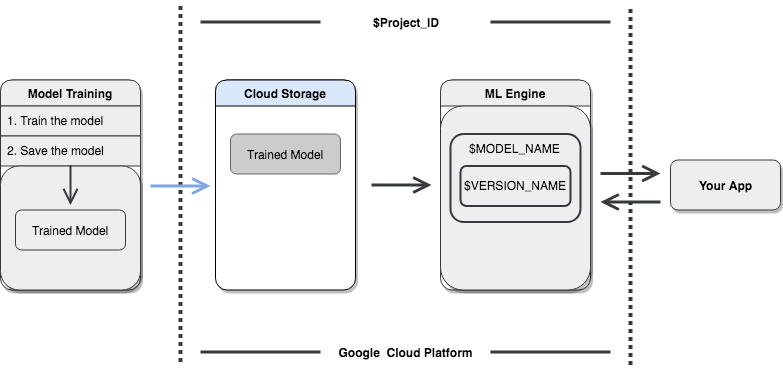To use your model with ML Engine, you’ll need to upload it to Google Cloud Storage. This step takes your local model.joblib file and uploads it to Cloud Storage via the Cloud SDK using gsutil. $ gsutil cp ./model.joblib $MODEL_PATH/model.joblib  ### Create a model resource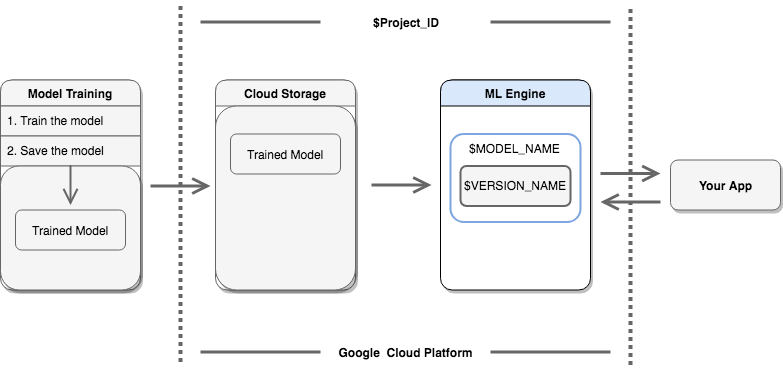Cloud ML Engine organizes your trained models using model and version resources. A Cloud ML Engine model is a container for the versions of your machine learning model. More information on model resources and model versions can be found in the scikit-learn documentation. In this step you’ll create a container that can hold several different versions of your actual model. $ gcloud ml-engine models create $MODEL_NAME --regions$REGION


### Create a model version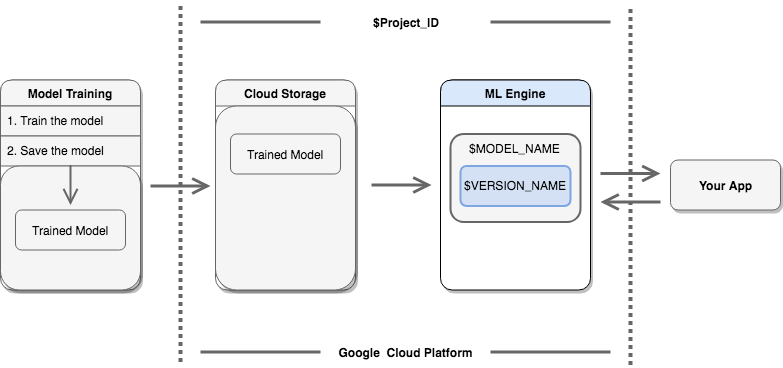Now it’s time to get a version of your model uploaded to your container. This will allow you to make online predictions. The model version requires a few components, specified here.

• name - The name specified for the version when it was created. This will be the VERSION_NAME variable you declared at the beginning.
• deploymentUri - The Google Cloud Storage location of the trained model used to create the version. This is the bucket where you uploaded the model with your MODEL_PATH.
• runtimeVersion - The Cloud ML Engine runtime version to use for this deployment. This is set to 1.4.
• framework - This specifies whether you’re using SCIKIT_LEARN or XGBOOST. This is set to SCIKIT_LEARN.
• pythonVersion - This specifies whether you’re using Python 2.7 or Python 3.5. The default value is set to “2.7”, if you are using Python 3.5, set the value to “3.5”

// WARNING: The gcloud ml-engine commands have been renamed and will soon be removed. Please use gcloud ai-platform instead
$gcloud beta ml-engine versions create$VERSION_NAME \
--model $MODEL_NAME \ --origin$MODEL_PATH \
--runtime-version="1.4" \
--framework="SCIKIT_LEARN" \
--python-version="3.5"


// You can check the status of your model's deployment with the following command:
$gcloud ml-engine versions list --model$MODEL_NAME


### Make an online prediction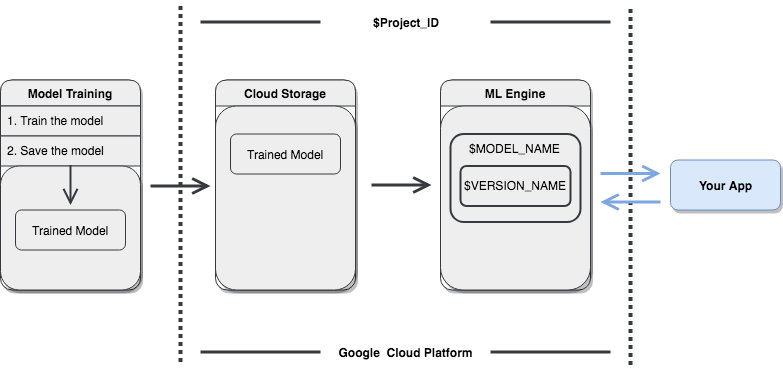It’s time to make an online prediction in Python with your newly deployed model.

$vi test.py  import googleapiclient.discovery import numpy as np import os import pandas as pd PROJECT_ID = os.environ['PROJECT_ID'] VERSION_NAME = os.environ['VERSION_NAME'] MODEL_NAME = os.environ['MODEL_NAME'] # Define the format of your input data including unused columns (These are the columns from the census data files) COLUMNS = ( 'age', 'workclass', 'fnlwgt', 'education', 'education-num', 'marital-status', 'occupation', 'relationship', 'race', 'sex', 'capital-gain', 'capital-loss', 'hours-per-week', 'native-country', 'income-level' ) # Categorical columns are columns that need to be turned into a numerical value to be used by scikit-learn CATEGORICAL_COLUMNS = ( 'workclass', 'education', 'marital-status', 'occupation', 'relationship', 'race', 'sex', 'native-country' ) # Load the training census dataset with open('./census_data/adult.data', 'r') as train_data: raw_training_data = pd.read_csv(train_data, header=None, names=COLUMNS) # Remove the column we are trying to predict ('income-level') from our features list # Convert the Dataframe to a lists of lists train_features = raw_training_data.drop('income-level', axis=1).as_matrix().tolist() # Create our training labels list, convert the Dataframe to a lists of lists train_labels = (raw_training_data['income-level'] == ' >50K').as_matrix().tolist() # Load the test census dataset with open('./census_data/adult.test', 'r') as test_data: raw_testing_data = pd.read_csv(test_data, names=COLUMNS, skiprows=1) # Remove the column we are trying to predict ('income-level') from our features list # Convert the Dataframe to a lists of lists test_features = raw_testing_data.drop('income-level', axis=1).as_matrix().tolist() # Create our training labels list, convert the Dataframe to a lists of lists test_labels = (raw_testing_data['income-level'] == ' >50K.').as_matrix().tolist() service = googleapiclient.discovery.build('ml', 'v1') name = 'projects/{}/models/{}'.format(PROJECT_ID, MODEL_NAME) name += '/versions/{}'.format(VERSION_NAME) # Due to the size of the data, it needs to be split in 2 first_half = test_features[:int(len(test_features)/2)] second_half = test_features[int(len(test_features)/2):] complete_results = [] for data in [first_half, second_half]: responses = service.projects().predict( name=name, body={'instances': data} ).execute() if 'error' in responses: print(response['error']) else: complete_results.extend(responses['predictions']) # Print the first 10 responses for i, response in enumerate(complete_results[:10]): print('Prediction: {}\tLabel: {}'.format(response, test_labels[i]))  $ python test.py


My output:

Prediction: False       Label: False
Prediction: False       Label: False
Prediction: False       Label: True
Prediction: True        Label: True
Prediction: False       Label: False
Prediction: False       Label: False
Prediction: False       Label: False
Prediction: True        Label: True
Prediction: False       Label: False
Prediction: False       Label: False


How did your model perform? Most likely the predictions and the labels are the same, indicating that the trained model performed well.